## Moore-Penrose Generalized Matrix Inverse

Given anMatrix, the Moore-Penrose generalized Matrix Inverse is a uniqueMatrixwhich satisfies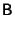(1)(2)(3)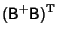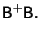(4)

It is also true that(5)

is the shortest length Least Squares solution to the problem(6)

If the inverse ofexists, then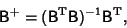(7)

whereis the Matrix Transpose, as can be seen by premultiplying both sides of (7) byto create a Square Matrix which can then be inverted,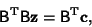(8)

giving(9)

References

Ben-Israel, A. and Greville, T. N. E. Generalized Inverses: Theory and Applications. New York: Wiley, 1977.

Lawson, C. and Hanson, R. Solving Least Squares Problems. Englewood Cliffs, NJ: Prentice-Hall, 1974.

Penrose, R. A Generalized Inverse for Matrices.'' Proc. Cambridge Phil. Soc. 51, 406-413, 1955.